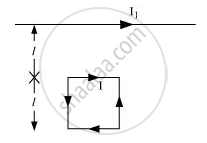# Write the Expression for the Magnetic Moment VecmDue to a Planar Square Loop of Side ‘L’ Carrying a Steady Current I in a Vector Form. - Physics

Write the expression for the magnetic moment vecmdue to a planar square loop of side ‘l’ carrying a steady current I in a vector form.

In the given figure this loop is placed in a horizontal plane near a long straight conductor carrying a steady current I1 at a distance l as shown. Give reason to explain that the loop will experience a net force but no torque. Write the expression for this force acting on the loop.#### Solution

The expression for the magnetic moment vecmdue to a planar square loop of side ‘l’ carrying a steady current I in a vector form is given as

vecm = IvecA

Therefore,

vecm = I(l)^2  hatn

Where, vecn is the unit vector along the normal to the surface of the loop.

The attractive force per unit length on the loop is

F_a = (mu_0)/(2pi) (I_1I)/I

F_a = (mu_0)/(2pi)I_1I

The repulsive force per unit length on the loop is

F_r/l  = (mu _0)/(2pi) (I_1I)/(2l)

F_r = (mu _0)/(2pi) (I_1I)/(2l)

F_(\text { net}) = F_a - F_t

= (mu_0 )/(2pi)I_1I(1-1/2)

|F_(text {net})  = (mu_0)/(4pi)I_1I|

Since the attractive force is greater than the repulsive force, a net force acts on the loop.

The torque on the loop is given as

tau = vecm xx vecB

=mBsintheta

=IAB sintheta

θ = 0° (becauseArea vector is parallel to the magnetic field)

τ = IAB sin0°

τ = 0

∴ The torque acting on the loop is zero.

Concept: Torque on a Current Loop in Magnetic Field
Is there an error in this question or solution?
Chapter 4: Moving Charges and Magnetism - Exercise 1

#### APPEARS IN

NCERT Class 12 Physics Textbook
Chapter 4 Moving Charges and Magnetism
Exercise 1 | Q 1

Share